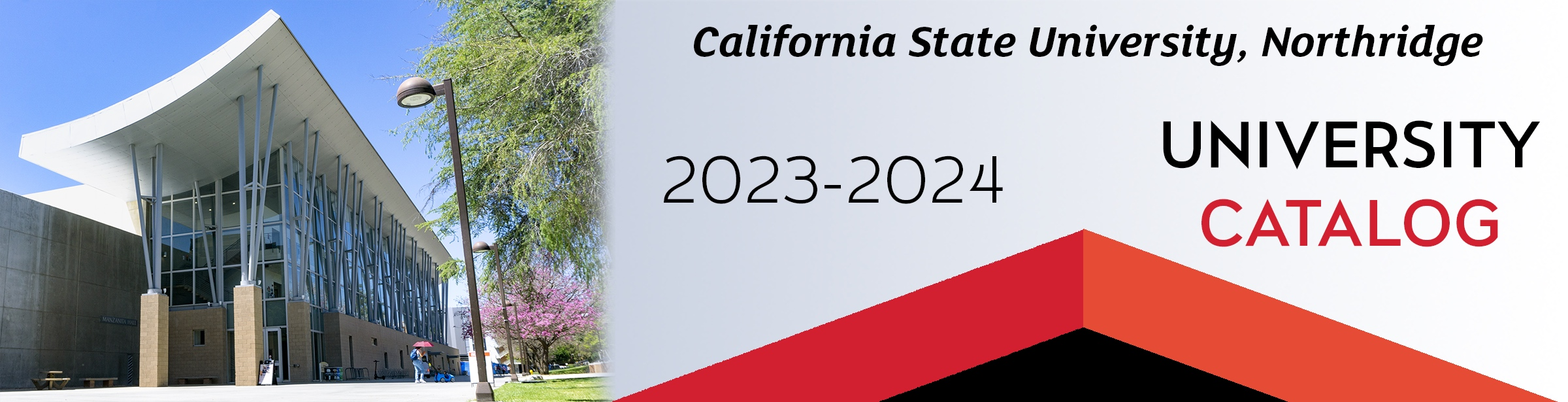## Course: MATH 545. Probability Theory (3)

Prerequisite: MATH 552. Operations on sets and events, sigma algebras, probability measures, Lebesgue measure, measurable maps and random variables, independence, Borel-Cantelli lemmas, zero-one laws, integration with respect to a probability measure, convergence theorems for integral, product spaces, and Fubini’s theorem. Laws of large numbers, convergence in distribution, and the central limit theorem.

### MATH 545

Class NumberLocationDayTime